### 2. BASIC CONCEPTS OF GRAVITATIONAL LENSING

2.1 The Thin Gravitational Lens

The standard gravitational lens theory is valid for weak stationary gravitational fields. For small angle deviations, and when the typical size of the deflector is much smaller than the propagation medium, it is possible to use the thin lens approximation (Blandford & Kochanek 1988). The lens is achromatic and acts as a plane of matter with a projected surface mass density(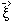).

The angular position of the sourceis related to the angular position of the image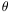through the lensing equation which expresses the simple geometrical relationship shown in Fig. 3: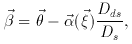(1)

with : Dds = angular diameter distance from deflector to source; Ds = angular diameter distance from observer to source.

For a ray crossing the lens with impact parameter, the deviation angle depends on the projected mass distribution(). More precisely, the deflection angle depends on the gradient of the 2D-potential in the deflector plane,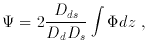(2)

whereis the three dimensional potential, which can be derived from() through the Poisson equation (3):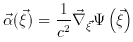(3)

with :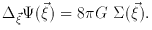(4)

A ray is deflected with the concavity of the trajectory oriented toward the local gravity field vector. The gradient of the potential plays a similar role as a gradient of index (1 - 2/ c2) in classical optics (Schneider, Ehlers & Falco 1992).

Using the magnification axes for the angular coordinates equation (1) can be written as :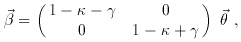(5)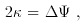(6)

and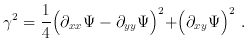(7)

The flux magnification µ is given by the determinant of the magnification tensor d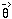/ d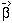: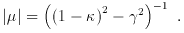(8)

If the shear parameteris zero, we have the equivalent of a uniform sheet of matter. Due to the local mass density, a small beam of light crossing the lens will have just an homothetic shrinking of its cross section by a factor ( 1 -)2. It can be see from expression (4) that an uniform sheet of matter focuses all the rays coming from a source onto the observer if= 1. This condition can be explicitly written as (Blandford & Kochaneck 1988; Schneider, Ehlers & Falco 1992):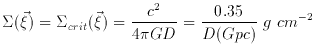(9)

with D = DdDds / Ds.

If the shearis non-zero the cross section will have an extra ellipticity induced by the differential deflection of the rays along the local gravity vector resulting from the total mass of the lens. This explains the formation of arclets and the weak statistical distortion of background galaxies observed at the periphery of clusters (Figs. 4 and 5).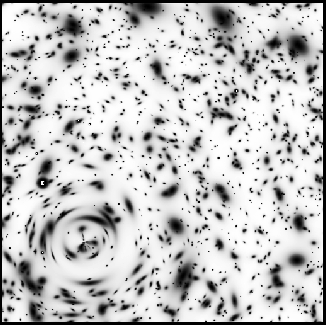Figure 5. Distortion field generated by a lens. The top panel shows the grid of randomly distributed background sources as it would be seen in the absence of the lens. The projected number density corresponds to the Tyson population at a limiting magnitude B = 28 (Tyson 1988). The bottom panel shows the same population once they are distorted by a foreground (invisible) circular cluster with a typical velocity dispersion of 1300 km s-1. The geometrical signature of the cluster is clearly visible. The potential can be recovered by using the formalism defined in part 4. In this simulation, the sources are at z = 1.3, and the cluster at z = 0.15.

Except for some unusual lenses, a general mass distribution() focuses multiple images onto the observer if its surface density exceedscrit at some point(see part 3 on marginal lenses). From expression (1) it can be seen that the deviation angle is proportional to a geometrical term that only depends on the angular distances of the deflector and sources. If the redshifts of the source and the lens are known, the astrometric positions of the images will depend on the geometry of the universe. This could be used as a tool for cosmography (cf part 5).

For gravitational images Etherington (1933) demonstrated that the local surface brightness of an image point is equal to the surface brightness of the antecedent point on the source viewed without the deflector. For an observer this has the deceiving consequence that the apparent surface brightness of objects is conserved : the contrast on the sky of faint distant galaxies will not be changed by the lens (cf sect. 3). But this theorem provides one of the best constraints for lens modeling (Kochanek et al. 1989, see sect. 3.4). However, an amplification of light is obtained from the geometrical magnification of the images. This is particularly interesting for spectroscopy of magnified sources. Note that for a point-like object like a QSO, the total amplification of light and the image position will be the only observable parameters.